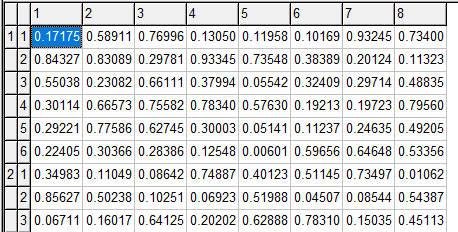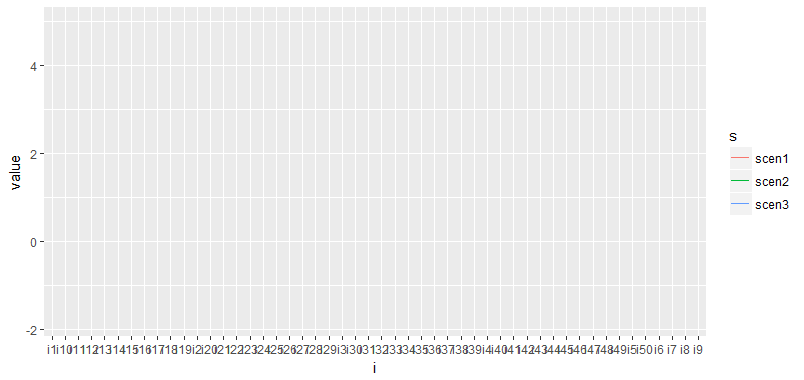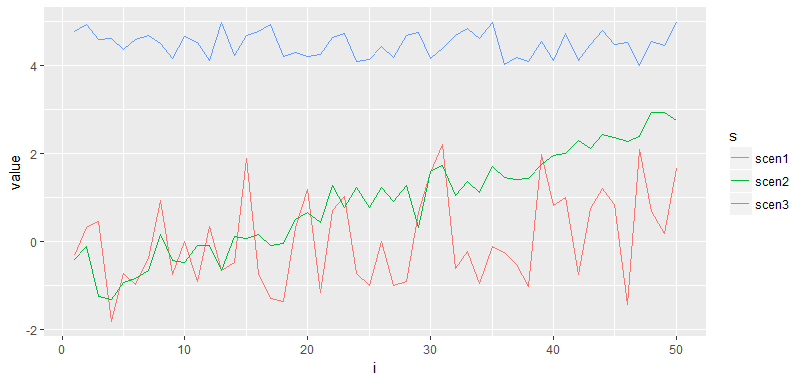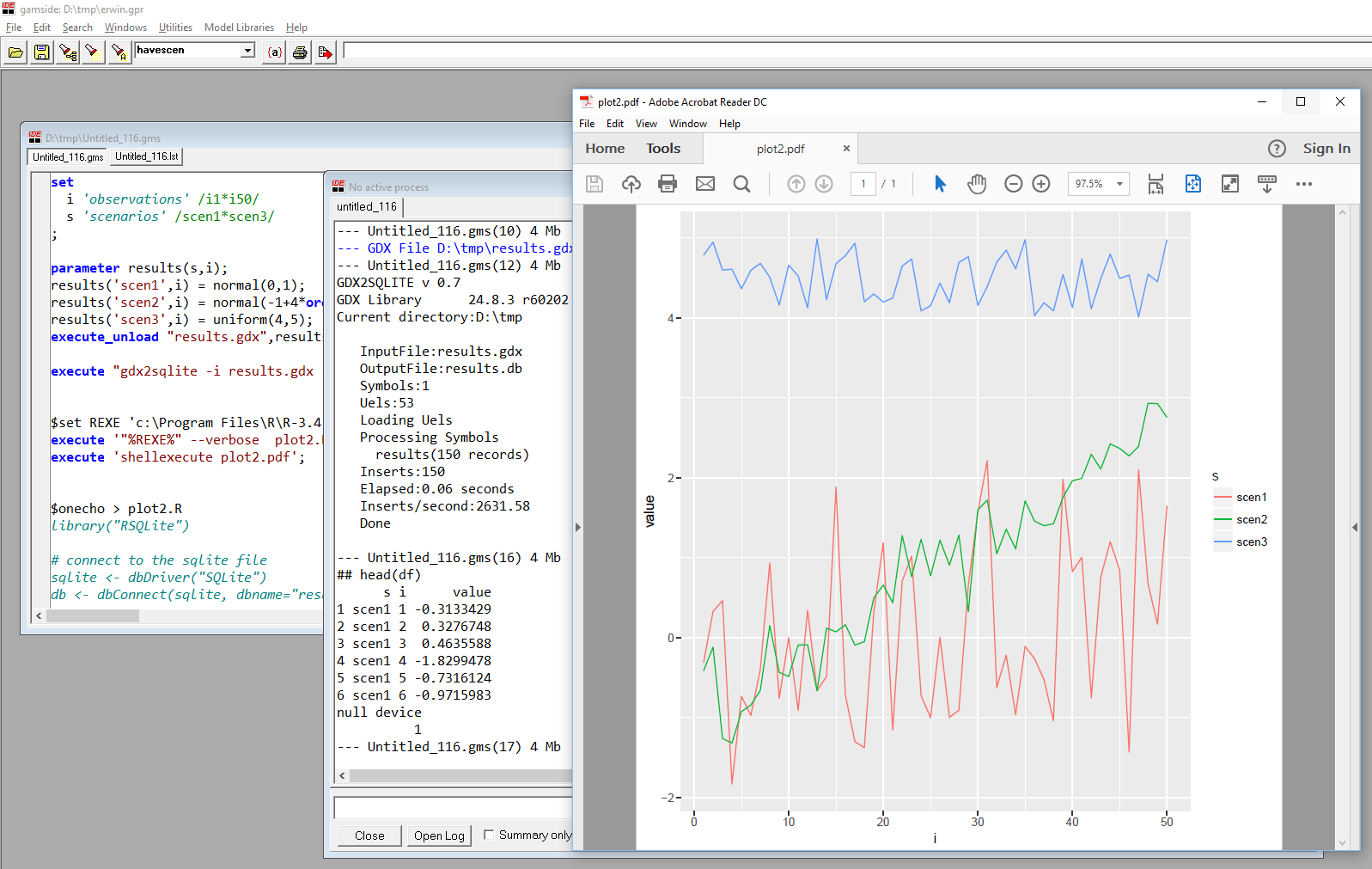## Thursday, August 10, 2017

### Element renaming

In GAMS set elements (used for indexing) are strings. This means it is not necessary to use non-descriptive numbers as in:

set i /1*50/;

Actually, even with numbered elements, it is good practice to prefix such elements, as in:

set i /i1*i50/;

This will help when inspecting large, multi-dimensional data-structures.

As an example consider the code fragment:

set
i /1*8/
j /1*4/
k /1*6/
;

parameter p(i,j,k);
p(i,j,k) = uniform(0,1);

I see these type of numbered sets quite often. Here is why this may be a bad idea: especially after pivoting a bit, it is really difficult to see what is going on:If we prefix the set elements with a name, we achieve much more clarity:When I receive a model with numbered set elements the first thing I try to do is to prefix the labels.

Of course, in practical models, we often can use meaningful names, but sometimes we just have numbers as IDs. I have also seen cases where the ID is a number or a very long description. In that case, the number can be a better candidate for set element.

### Exporting set elements

In GAMS a parameter is really a sparse (multi-dimensional) matrix. This corresponds quite nicely to a table in a RDBMS (relational database) or to a dataframe in R or Python. A matrix in R or Python is dense, so those data structures do not work as well for large, sparse parameters, although R has nice facilities to use matrices with row and column names.

### Example

Let’s generate some data in GAMS and export to an SQLite database:

set
i 'observations' /i1*i50/
s 'scenarios' /scen1*scen3/
;

parameter results(s,i);
results('scen1',i) = normal(0,1);
results('scen2',i) = normal(-1+4*ord(i)/card(i),0.4);
results('scen3',i) = uniform(4,5);
execute_unload "results.gdx",results;

execute "gdx2sqlite -i results.gdx -o results.db";

We can try to plot this data in R as follows:

```library("RSQLite")
library("ggplot2")

# connect to the sqlite file
sqlite = dbDriver("SQLite")
db = dbConnect(sqlite, "results.db")

# retrieve data
df = dbGetQuery(db,"select * from results")
head(df)

ggplot(data=df,aes(x=i,y=value,color=s)) + geom_line()

dbDisconnect(db)
```

The output of head(df) indicates we read the data correctly:

## head(df)
s  i      value
1 scen1 i1 -0.3133429
2 scen1 i2  0.3276748
3 scen1 i3  0.4635588
4 scen1 i4 -1.8299478
5 scen1 i5 -0.7316124
6 scen1 i6 -0.9715983
Unfortunately, things don’t work exactly as expected. We see an error message:
geom_path: Each group consists of only one observation. Do you need to adjust
the group aesthetic?

And the plot does not look correct:The reason is the variable df\$i.If we convert this back to integers we are ok. To do this conversion we actually have to do two things:

1. Drop the first character ‘i’ from df\$i.
2. Convert df\$i from string to numeric (type casting).

Interestingly we can fix this in several stages:

### Partial GAMS solution

We can not really rename set elements in GAMS. However we can create a new parameter, a mapping set and use a sum statement to map between sets:
set
i 'observations' /i1*i50/
s 'scenarios' /scen1*scen3/
i2 'observations as number' /1*50/
map(i2,i) '1-1 mapping set'
;
map(i2,i)\$(ord(i2)=ord(i)) = yes;

parameter results(s,i);
results('scen1',i) = normal(0,1);
results('scen2',i) = normal(-1+4*ord(i)/card(i),0.4);
results('scen3',i) = uniform(4,5);

parameter results2(s,i2);
results2(s,i2) = sum(map(i2,i),results(s,i));

Now we can export the parameter results2 instead of results. Note that index i2 will still be a string when it arrives in R. The type casting to a numeric type has to be done in R or in SQL (see next sections how this can be done).

### Conversion in R

The conversion to a numeric type can be done in R in just one line

```df\$i <- as.numeric(substring(df\$i,2))
```
Now things look better:### Conversion in SQL

We can also perform the conversion in SQL while reading the data from the SQLite database file:

select s,
cast(substr(i,2) as numeric) as i,
value
from results

### Scripting

This whole thing can be easily scripted in GAMS.